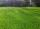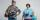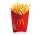# Basic functions - math word problems

#### Number of problems found: 2468

• Six on diceWhat is the probability that when throwing two dice will fall at least one six?
• Isosceles triangleThe perimeter of an isosceles triangle is 112 cm. The length of the arm to the length of the base is at ratio 5:6. Find the triangle area.
• Sum or productWhat is the probability that two dice fall will have the sum 7 or product 12?
• Cube surface areaThe surface of the cube was originally 216 centimeters square. The surface of the cube has shrunk from 216 to 54 centimeters sq. Calculate how much percent the edge of the cube has decreased.
• Mow the lawnDano would mow the lawn in 12 hours and Milada in 16 hours. How long will take the lawn mow together?
• LinesHow many lines can be draw with 8 points, if three points lie on one line and the other any three points do not lie on the same line?
• Three-digitHow many do three-digit natural numbers not have the number 7?
• DigitsHow many five-digit numbers can be written from numbers 0.3,4, 5, 7 that is divided by 10, and if digits can be repeated?
• Book readIf Petra read 10 pages per day, she would read the book two days earlier than she read 6 pages a day. How many pages does a book have?
• Ratio of two unknown numbersTwo numbers are given. Their sum is 30. We calculate one-sixth of a larger number and add to both numbers. So we get new numbers whose ratio is 5:7. Which two numbers were given?
• Direct routeFrom two different places A and B connected by a direct route, Adam (from city A) and Bohus (from city B) started at a constant speed. As Adam continued to go from A to B, Bohus turned around at the time of their meeting and at the same speed he returned
• Mason with assistantMason had to complete the work in 10 days. Two and a half days later, his assistant arrived. Together they completed the work in the next three and a half days. How many days would he need an assistant for the same work?
• Odd numbersThe sum of four consecutive odd numbers is 1048. Find those numbers ...
• Cars speedsDistance from city A to city B is 108 km. Two cars were simultaneously started from both places. The speed of a car coming from city A was 2 km/h higher than the second car. What was the speed of each car if they met in 54 minutes?
• TestingStudents of high school have 10 points for each good solved task. The wrong answer is deducted by 5 points. After solving 20 tasks, student Michael had 80 points. How many tasks did he solve correctly and how many wrong?
• Boys and girls 2The ratio of boys to girls in math club is 4:3 . After 8 more girls joined the Club, the ratio become 1:1. How many members are there in the club now?
• Unknown number 24f we add 20, we get 50% of its triple. What is this unknown number?
• Parcel 4Send a parcel by messenger within city limits costs 60 cents for the first pound and 48 cents for each additional pound. What is the cost, in cents, of sending a parcel weighing p=4 pounds?
• GarageThere are two laths in the garage opposite one another: one 2 meters long and the second 3 meters long. They fall against each other and stay against the opposite walls of the garage and both laths cross 70 cm above the garage floor. How wide is the garag
• CaloriesA large order of McDonald’s french fries has 500 calories. Of this total, 220 calories are from fat. Find the ratio of the calories from fat to total calories in a large order of McDonald’s french fries.

Do you have an interesting mathematical word problem that you can't solve it? Submit a math problem, and we can try to solve it.

We will send a solution to your e-mail address. Solved examples are also published here. Please enter the e-mail correctly and check whether you don't have a full mailbox.

Please do not submit problems from current active competitions such as Mathematical Olympiad, correspondence seminars etc...